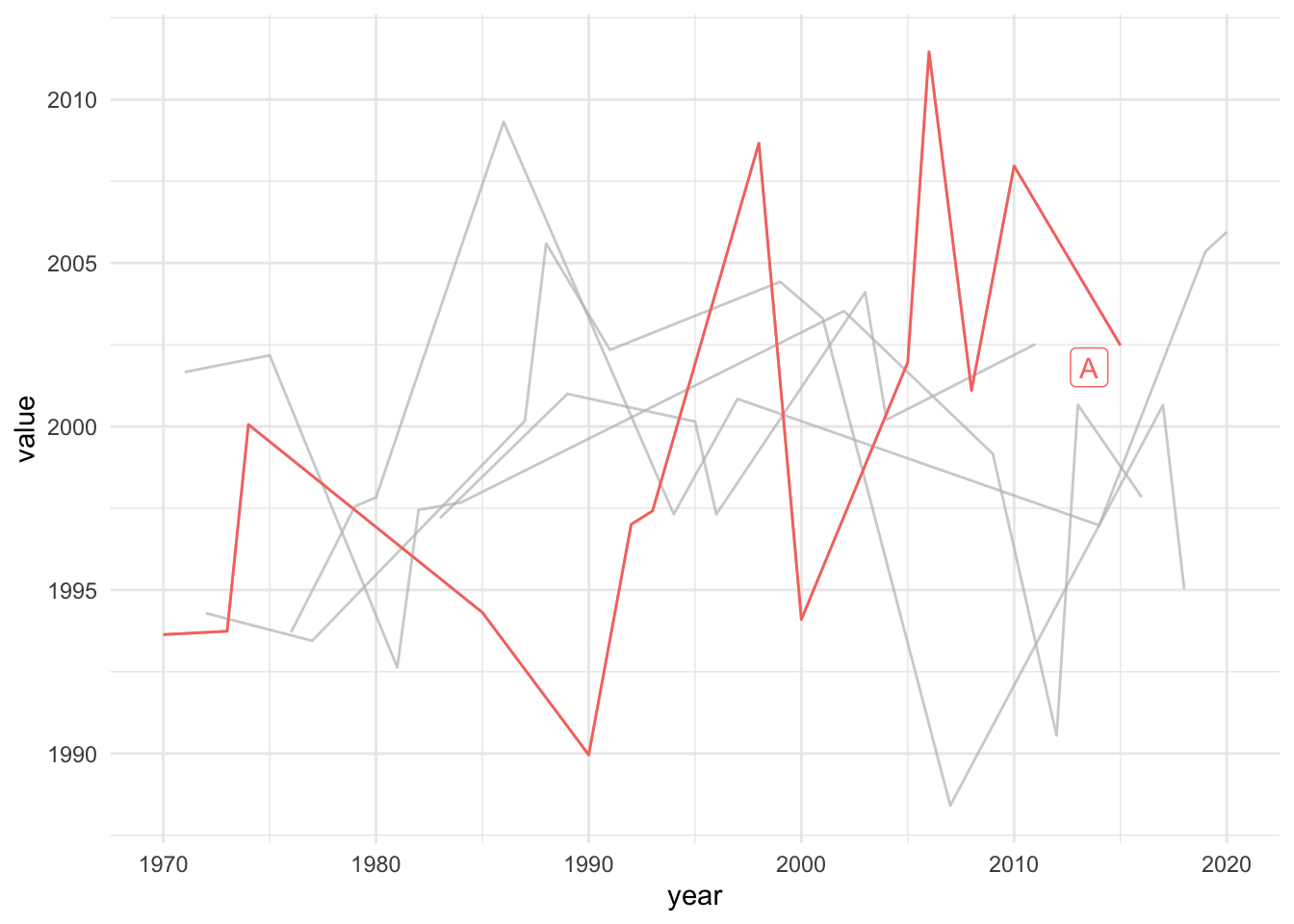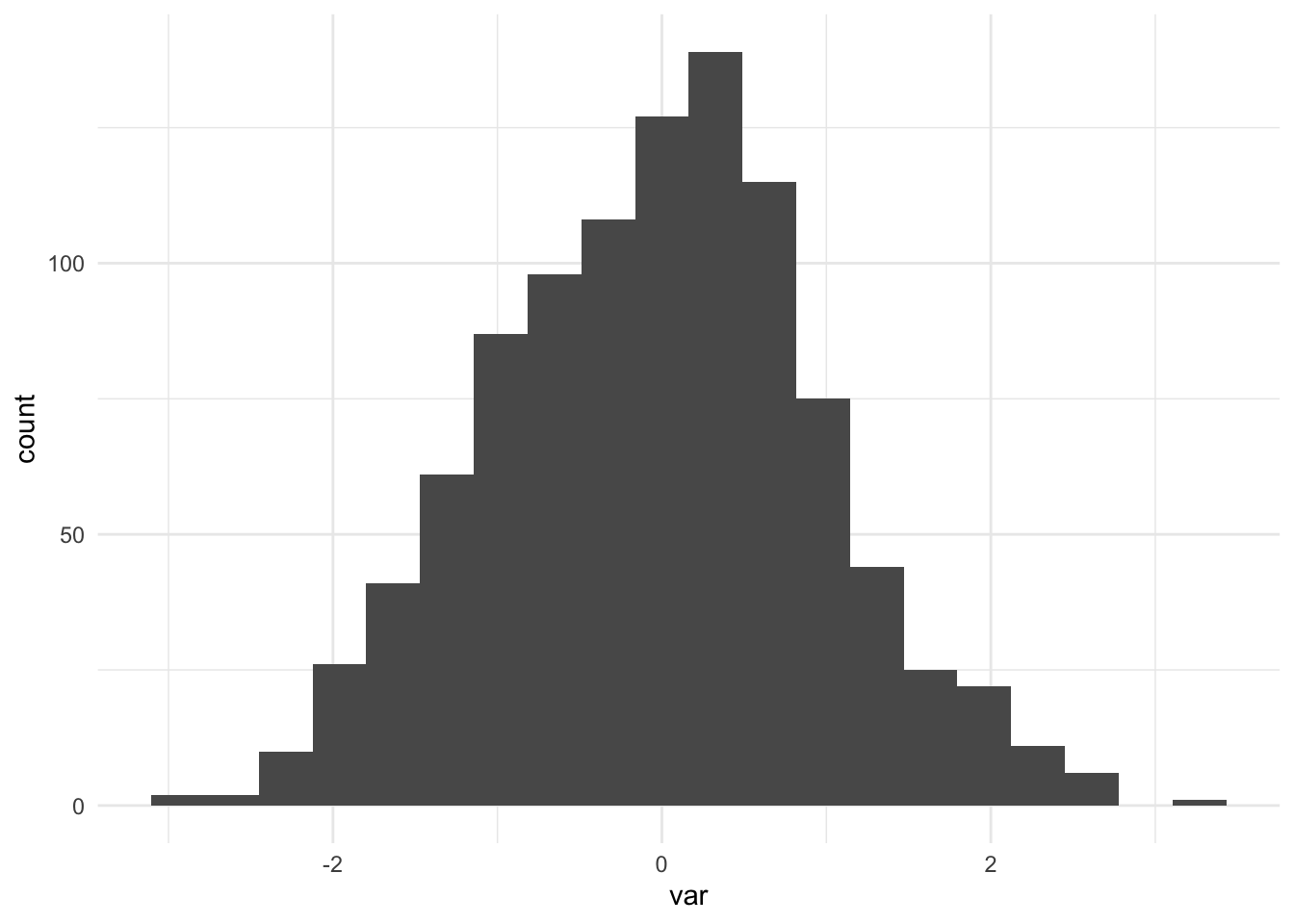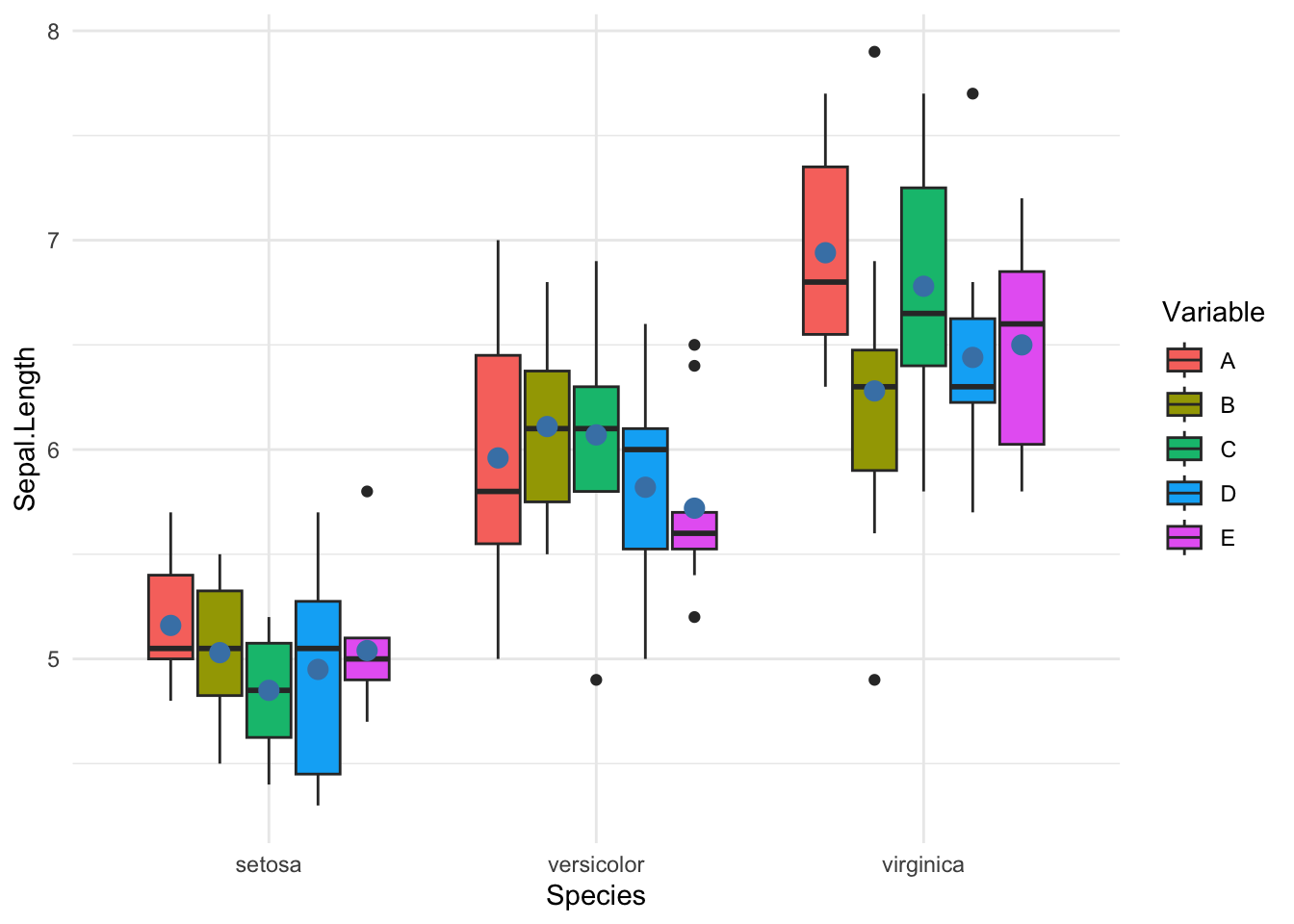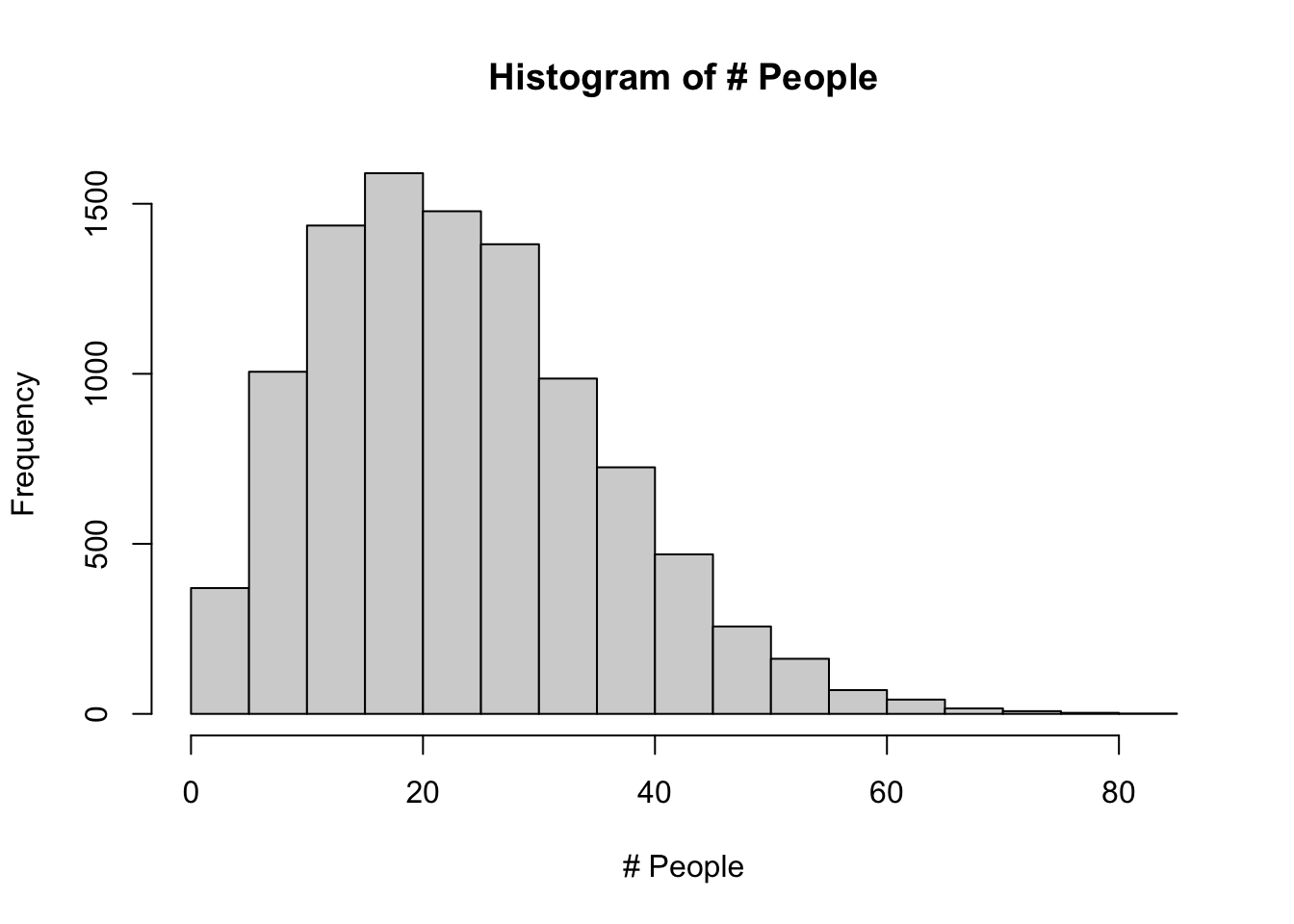Most of the time when I answer questions on Stack Overflow, I end up learning a thing or two about R myself. Answering questions gets me warmed up on unrelated topics. These questions are one of their kind. This blog post documents all my answers so that I can find the answers readily.

The headlines explain the basic logic of what I am trying to achieve through that question.

## Visualisation

### This question was on combining time series plots using par().

par() won’t work because plot.decomposed.ts() (which we implicitly call when calling plot()) isn’t designed to work that way. The most straightforward alternative is to use autoplot() from the forecast package to generate decomposition plots and combine them using patchwork.

Here is an example.

# Loading forecast and patchwork
library(forecast)

## Registered S3 method overwritten by 'quantmod':
##   method            from
##   as.zoo.data.frame zoo

library(patchwork)

m1 = decompose(co2)
m2 = decompose(AirPassengers)
m3 = decompose(UKgas)
p1 = autoplot(m1)
p2 = autoplot(m2)
p3 = autoplot(m3)
p1 / p2 / p3The last line, p1 / p2 / p3, tells R to stack them vertically. If you want to stack them horizontally, use p1 + p2 + p3. If you’re being feisty, you can also try (p1 + p2)/p3 to stack the first two horizontally and the last one beneath it.

### Usinggghighlight to highlight a line plot in ggplot2

gghighlight provides gghighlight() which can be used to selectively highlight some lines, points or other geom_. I couldn’t get the dataset in question working, so I generated a random dataset. The code should work for their case as well.

library(gghighlight)

## Loading required package: ggplot2

year = 1970:2020
value = rnorm(length(year), 2000, 5)
x = c("A", "B", "C", "D", "E")
variable = sample(x, length(year), replace = T)
df = data.frame(year = year,
value = value,
variable = variable)


Now is the cool part.

df %>%
ggplot(aes(x = year, y = value, colour = variable)) +
geom_line() +
gghighlight(variable == "A") +
theme_minimal()

## Warning: Tried to calculate with group_by(), but the calculation failed.
## Falling back to ungrouped filter operation...

## Warning: Using across() in filter() is deprecated, use if_any() or
## if_all().

## label_key: variableVoila!

### Histogram with ggplot2

I had to clarify several things. First, what made them choose bins = 43? Second, is providing the scale manually necessary? If the data is in the right format, they should not need it. If it isn’t, they should first transform the data and then do it.

Third, gray background (which they wanted to change) is from the default theme. There are several options but I like minimal. minimal, linedraw and bw have white grids.

(I’m generating 1000 random numbers for this example.)

library(tidyverse)

## ── Attaching packages ─────────────────────────────────────── tidyverse 1.3.1 ──

## ✓ tibble  3.1.6          ✓ dplyr   1.0.7.9000
## ✓ tidyr   1.1.4          ✓ stringr 1.4.0
## ✓ readr   2.0.2          ✓ forcats 0.5.1
## ✓ purrr   0.3.4

## ── Conflicts ────────────────────────────────────────── tidyverse_conflicts() ──

v = tibble(var = rnorm(1000))
ggplot(v, aes(x = var)) +
geom_histogram(bins = 20) +
theme_minimal()### Barplots grouped by two (factor) variables and plotting mean values as points on them

The data they posted didn’t work so I’ve used (modified) iris dataset.

# loading tidyverse
library(tidyverse)

# adding another factor variable to replicate this example
iris$Variable = rep(LETTERS[1:5], times = 30)  Here’s the meat. iris %>% ggplot(aes(x = Species, y = Sepal.Length, fill = Variable)) + geom_boxplot() + stat_summary( fun = mean, color = "steelblue", position = position_dodge(0.75), geom = "point", shape = 20, size = 5, show.legend = FALSE ) + theme_minimal()## String Manipulations ### Selecting rows where a string match occurs We can use grepl() from base R for this. grepl() returns True if the word is present and False otherwise. text = "The Little Vanities of Mrs. Whittaker: A Novel" word = "Novel" grepl(word, text)  ##  TRUE  The original_books file (in question) will require large downloads so I’m showing an example of searching “Plays” in title.x of their novels data frame. library(gutenbergr) library(tidyverse) gutenberg_full_data <- left_join(gutenberg_works(language == "en"), gutenberg_metadata, by = "gutenberg_id") gutenberg_full_data <- left_join(gutenberg_full_data, gutenberg_subjects)  ## Joining, by = "gutenberg_id"  gutenberg_full_data <- subset( gutenberg_full_data, select = -c( rights.x, has_text.x, language.y, gutenberg_bookshelf.x, gutenberg_bookshelf.y, rights.y, has_text.y, gutenberg_bookshelf.y, gutenberg_author_id.y, title.y, author.y ) ) gutenberg_full_data <- gutenberg_full_data[-which(is.na(gutenberg_full_data$author.x)), ]
novels <- gutenberg_full_data %>% filter(subject == "Drama")


Here comes the cool part.

novels %>%
mutate(contains_play = grepl("Plays", title.x)) %>%
as.data.frame() %>%

##   gutenberg_id                                        title.x
## 1         1308     A Florentine Tragedy; La Sainte Courtisane
## 2         2270                      Shakespeare's First Folio
## 3         2587                                Life Is a Dream
## 4         4970                    There Are Crimes and Crimes
## 5         5053 Plays by August Strindberg: Creditors. Pariah.
## 6         5618                                      Six Plays
##                                  author.x gutenberg_author_id.x language.x
## 1                            Wilde, Oscar                   111         en
## 2                    Shakespeare, William                    65         en
## 3             Calderón de la Barca, Pedro                   970         en
## 4                      Strindberg, August                  1609         en
## 5                      Strindberg, August                  1609         en
## 6 Darwin, Florence Henrietta Fisher, Lady                  1814         en
##   subject_type subject contains_play
## 1         lcsh   Drama         FALSE
## 2         lcsh   Drama         FALSE
## 3         lcsh   Drama         FALSE
## 4         lcsh   Drama         FALSE
## 5         lcsh   Drama          TRUE
## 6         lcsh   Drama          TRUE


Note that grepl() allows the second argument to be a vector. Thus, using rowwise() is not necessary. If it allowed searching only within a string, we would have to use rowwise().

## Data Wrangling and Manipulation

### Typecasting to Numeric

The variable born is registered as a character variable. Convert it to numeric and one should be good to go.

dat1$born = as.numeric(dat1$born)


Now compute the age difference.

### Grabbing columns from one data frame with variable names from another data frame

One can do it using any_of() function from dplyr. It selects the variables which match the names and ignores those which do not. I will use a list to store matrices from the loop. They can be accessed using df_modified[[i]].

df1=data.frame(q1 = c(1:3),
q2 = c("One" , "Two" , "Three") ,
q3 = c(100,231,523),
q4 = c("red", "green", "blue"),
q1.2 = c(20:22),
q2.2 = c("Six" , "Ten" , "Twenty") ,
q3.2 = c(5,900,121),
q4.2 = c("purple", "yellow", "white"))
df2=data.frame(x1 = c("q1" , "q2.1" , "q3.2" , "q4.2") ,
x2 = c("q2" , "q3" , "q3.3" , "q4.4") ,
x3 = c("q3" , "q2.4" , "q3.3" , "q4.6"),
x4 = c("q4" , "q3.6" , "q3.3" , "q4.2"))

# Loading libraries
library(tidyverse)

df_modified = list()
for(i in 1:nrow(df2))
{
vars = as.character(df2[i,])
df_modified[[i]] = df1 %>%
select(any_of(vars))
}


Output

df_modified

## []
##   q1    q2  q3    q4
## 1  1   One 100   red
## 2  2   Two 231 green
## 3  3 Three 523  blue
##
## []
##    q3
## 1 100
## 2 231
## 3 523
##
## []
##   q3.2
## 1    5
## 2  900
## 3  121
##
## []
##     q4.2
## 1 purple
## 2 yellow
## 3  white


Done!

### Selecting Observations by Filtering Other Variables

One approach is to write a function that does that for you. It matches the first three variables with what you input and returns the index(or indexes) of elements that match.

which() returns the index of items that satisfy the condition. When I say which(df[,1] == a), it will return me the index of observations in df where the first column matches a. And so on. Then, you can use intersect() to find the common indexes in x1, x2 and x3. I’m using magrittr pipes %>% to simplify the coding.

check_this = function(df, a, b, c)
{
x1 = which(df[,1] == a)
x2 = which(df[,2] == b)
x3 = which(df[,3] == c)

v = intersect(x1, x2) %>%
intersect(x3)
return(v)
}


Minimum Working Example First, I’ll create a dummy data frame. Then, I’ll find the index using the function I just created.

df = tibble(var1 = 1:10,
var2 = 11:20,
var3 = letters[1:10],
var4 = LETTERS[1:10])

df

## # A tibble: 10 × 4
##     var1  var2 var3  var4
##    <int> <int> <chr> <chr>
##  1     1    11 a     A
##  2     2    12 b     B
##  3     3    13 c     C
##  4     4    14 d     D
##  5     5    15 e     E
##  6     6    16 f     F
##  7     7    17 g     G
##  8     8    18 h     H
##  9     9    19 i     I
## 10    10    20 j     J


Now, let us see it in action. First, I’ll pass the data frame and variables I want to match as arguments. The function will return the indices which I’ll store in l. Then, I’ll ask R to show me the rows which have indices numbers in l.

# checking and storing the index of matched
l = check_this(df, 2, 12, "b")

df[l,]

## # A tibble: 1 × 4
##    var1  var2 var3  var4
##   <int> <int> <chr> <chr>
## 1     2    12 b     B


Note: You could have skipped the step of storing indices in l by returning the selected rows of the data frame itself. The function would change to the following.

# the function
check_this = function(df, a, b, c)
{
x1 = which(df[,1] == a)
x2 = which(df[,2] == b)
x3 = which(df[,3] == c)

v = intersect(x1, x2) %>%
intersect(x3)
return(df[v,])
}


### Convert a vector of strings in multiple formats into dates in R

#### My Solution

# sample date
dates <- c("2015-02-23","2015-02-12","2015-18-02","2015-25-02")

# libraries
library(testit) #for has_warning
library(lubridate) #for date functions

##
## Attaching package: 'lubridate'

## The following objects are masked from 'package:base':
##
##     date, intersect, setdiff, union


This function will correct the dates.

correct_dates = function(dates)
{
dates_new = character()
for(i in 1:length(dates))
{
#print(i)
if(has_warning(day(ydm(dates[i]))>12))
{dates_new = append(dates_new, ymd(dates[i]))}
else
{dates_new = append(dates_new, ydm(dates[i]))}
}
return(dates_new)
}


Let’s see it in action.

dates

##  "2015-02-23" "2015-02-12" "2015-18-02" "2015-25-02"

correct_dates(dates)

##  "2015-02-23" "2015-12-02" "2015-02-18" "2015-02-25"


#### Much Better Solution

dates <- c("2017-12-31","2017-12-30","2017-29-12","2017-28-12")
as.Date(lubridate::parse_date_time(dates, c('ymd', 'ydm')))

##  "2017-12-31" "2017-12-30" "2017-12-29" "2017-12-28"


## Table Formatting in kableExtra

### How to append two tables with same number of columns in kableExtra?

I don’t know how to combine the tables directly without first joining the data frames. However, using pack_rows to specify rows for grouping together should work for this purpose.

library(kableExtra)

##
## Attaching package: 'kableExtra'

## The following object is masked from 'package:dplyr':
##
##     group_rows

df1 = data.frame(x = c("a","b"), y=1:2)
df2 = data.frame(x = c("c","d"), y=3:4)

rbind(df1, df2) %>%
kbl(format = "latex", caption = "Combined Tables") %>%
kable_paper("striped", full_width = F) %>%


\begin{table}

\caption{(#tab:unnamed-chunk-21)Combined Tables} \centering \begin{tabular}[t]{l|r} \hline x & y\ \hline \multicolumn{2}{l}{\textbf{Header 1}}\ \hline \hspace{1em}a & 1\ \hline \hspace{1em}b & 2\ \hline \multicolumn{2}{l}{\textbf{Header 2}}\ \hline \hspace{1em}c & 3\ \hline \hspace{1em}d & 4\ \hline \end{tabular} \end{table}

Check the documentation of ?pack_rows from kableExtra to modify the group labels, add \hlines, or other such cosmetic changes.## Simulation

### How many people are needed such that there is at least a 70% chance that one of them is born on the last day of December?

The question is, “How many people are needed such that there is at least a 70% chance that one of them is born on the last day of December?”. What they were finding now is “How many people are needed such that 70% have their birthdays on the last day of December?”. The answer to the second question is close to zero. But the first one is much simpler.

Replacing prob <- length(which(birthday == 365)) / people with check = any(birthday == 365) in their logic because at least one of them has to be born on Dec 31 will work. Then, they will be able to find if that number of people will have at least one person born on Dec 31.

After that, they will have to rerun the simulation multiple times to generate empirical probability distribution (kind of Monte Carlo). Only then they can check for probability.

#### Simulation Code

people_count = function(i)
{
set.seed(i)
for (people in 1:10000)
{
birthday = sample(365, size = people, replace = TRUE)
check = any(birthday == 365)
if(check == TRUE)
{
pf = people
break
}
}
return(pf)
}


people_count() function returns the number of people required to have so that at least one of them was born on Dec 31. Then I rerun the simulation 10,000 times.

# Number of simulations
nsim = 10000
l = lapply(1:nsim, people_count) %>%
unlist()


Let’s see the distribution of the number of people required.

hist(l, main = "Histogram of # People",
xlab = "# People")To find actual probability, I’ll use cumsum().

cdf = cumsum(l/nsim)
which(cdf>0.7)

##  292


So, on average, you would need 292 people to have more than a 70% chance.

Posted on:
January 14, 2022
Length: